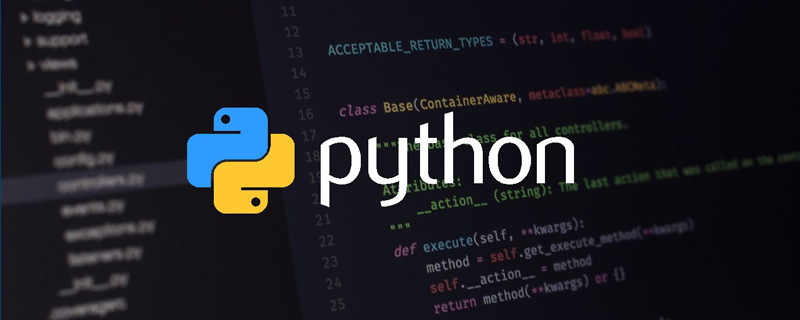# python怎么保留两位小数### 使用字符串格式化

```a = 12.345
print("%.2f" % a) # %代表格式化输出，.2代表小数点后保留两位，f代表数据类型是浮点型```

### 使用round内置函数

python内置了一个名为round的函数，这个函数可以用来对数据进行格式化。代码如下：

```a = 12.345
a1 = round(a, 2) #将a通过round函数处理后赋值给a1，传入的2代表保留两位小数
print(a1)```

### 使用decimal模块

```from decimal import Decimal
a = 12.345
Decimal(a).quantize(Decimal("0.00")) # 使用默认的进位方式（同round）“0.00”表示保留小数点后两位```

### 使用序列切片

```a = 12.345
str(a).split('.') + '.' + str(a).split('.')[:2] #使用序列切片的方式，切出小数点前的数据，还有小数点后两位的数据，然后进行拼装```

### 使用re模块

```import re
a = 12.345
re.findall(r"\d{1,}?\.\d{2}", str(a)) #使用正则匹配，将符合规则（一个小数点前有很多位数字，小数点后面只有两位数字的片段）字符串片段取出```

【相关推荐：Python3视频教程1/1